# Introduction to Cryptographic BasicsCryptography is the practice and study of techniques for secure communication in the presence of third parties. More generally, it is about constructing and analyzing protocols that overcome the influence of third parties and which are related to various aspects in information security such as data confidentiality, data integrity, authentication, and non-repudiation. Modern cryptography intersects the disciplines of mathematics, computer science, and electrical engineering. Applications of cryptography include ATM cards, computer passwords, and electronic commerce. In this article I introduce the Symmetry Law which can be useful for symmetric encryption of information.

The Symmetry Law

The symmetry of the set of the encrypted information is the characteristic that separates the set of such ciphered information into two identical or symmetric or mirrored parts. In binary logics, the symmetry is the property of XOR and XNOR functions. Some algorithms based on these two functions can produce the symmetric result. In the coming theorems all this will be explained and proved.

Firstly, let start with the logic function XOR and XNOR and let prove their symmetry.

Later, I will show how some ciphering algorithms based on these two functions or their combinations can be used for constructing the set of symmetric encrypted information.

Exclusive-OR or XOR function

Exclusive-OR or XOR function can be defined as follows. A true output (1) results if one, and only one, of the inputs to the gate is true (1). On the other hand, if both inputs are false (0) or both are true (1), a false output (0) results. Its behavior is summarized in the truth table. A way to remember XOR is “one or the other but not both”.

The truth table of XOR function: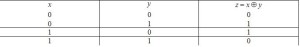The logic gate of XOR function is given in Figure 1.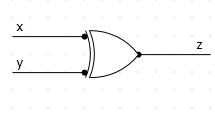Figure 1. XOR gate drawn in Logic Circuit software

As it is obvious from the truth table, an XOR function gives a set of symmetric output results for the different input combinations. That leads to conclusion that the XOR function is a symmetric function.

Exclusive-NOR or XNOR function

Exclusive-NOR or XNOR function is the inverse of the exclusive-OR or XOR function. It behaves according to its truth table. A high output (1) results if both of the inputs to the gate are the same. On the other hand, if one but not both inputs are high (1), a low output (0) results.

The truth table of XNOR function: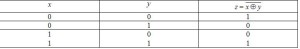The logic gate of XNOR function is shown in Figure 2.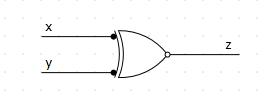Figure 2. XNOR gate designed in Logic Circuit software

Theorem 1:

Let assume that we observe a set of a-bit binary information, where a ≥ 3. The set of information contains different combinations of 0s and 1s. In that case, the source message can be represented using the weight coefficients. This is given in Equation (1).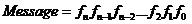Where:

n – the highest value of the weight coefficient for the message; n ≥ 2,

f – the weight coefficient for the message.

Let also assume that an encrypted message or a cipher can be presented as array of the weight coefficients, where number of digits for the message and number of digits for the cipher are the same. This is shown in Equation (2).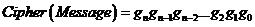Where:

n – the highest value of the weight coefficient for the cipher; n ≥ 2,

g – the weight coefficient for the cipher.

If the following algorithm (3) is applied, as a result the symmetric or mirrored set of encrypted or ciphered information will be gotten.The Proof:

The law will be proved using the case of the set of 4-bit binary information.

The set of 4-bit binary information:

Let observe the set of 4-bit binary information, which consists of 4 bits of information and has 2ª = 16 combinations. In this case, a number of bits or digits is a = 4. These combinations cover the set of the following decimal numbers: from 0 to 2ª – 1 = 15. All these combinations are given in Table 1 as follows.

Table 1.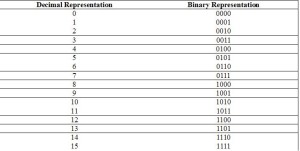Solution:

The results of such a provided ciphering process are given in Table 2. It is obvious that the set of the encrypted information is completely symmetric or mirrored, which is the consequence of the symmetric nature of XOR logic function. Table 2 is shown as follows.

Table 2.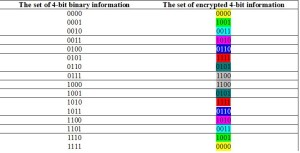Theorem 2:

Let assume that we observe a set of a-bit binary information, where a ≥ 3. The set of information contains different combinations of 0s and 1s. In that case, the source message can be represented using the weight coefficients. This is given in Equation (3).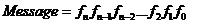Where:

n – the highest value of the weight coefficient for the message; n ≥ 2,

f – the weight coefficient for the message.

Let also assume that an encrypted message or a cipher can be presented as array of the weight coefficients, where number of digits for the message and number of digits for the cipher are the same. This is shown in Equation (4).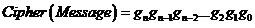Where:

n – the highest value of the weight coefficient for the cipher; n ≥ 2,

g – the weight coefficient for the cipher.

If the following algorithm (5) is applied, as a result the symmetric or mirrored set of encrypted or ciphered information will be gotten.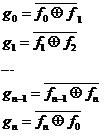The Proof:

The law will be proved using the case of the set of 4-bit binary information.

The set of 4-bit binary information:

Let observe the set of 4-bit binary information, which consists of 4 bits of information and has 2ª = 16 combinations. In this case, a number of bits or digits is a = 4. These combinations cover the set of the following decimal numbers: from 0 to 15. All these combinations are given in Table 3 as follows.

Table 3.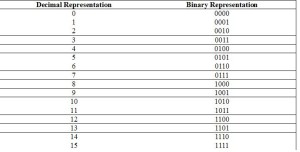The results of such a provided ciphering process are given in Table 4. It is obvious that the set of the encrypted information is completely symmetric or mirrored, which is the consequence of the symmetric nature of XNOR logic function. Table 4 is illustrated as follows.

Table 4.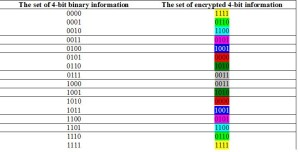Conclusions

This article provides a brief review of the results the author got during her research. The author plans to continue her research and to publish her findings into this magazine. The initial step has been made and the facts about the Symmetry Law have been shared with the audience. This is not the final version of the law, only the initial step. As research goes further, the author will be that free to publish the results.

Reference:

1.   Digital Systems – Principles and Applications, Ronald J. Tocci & Neal S. Widmer, Prentice-Hall International, Inc., 1998.